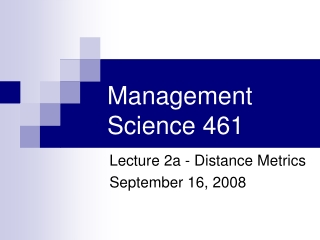DownloadDownload PresentationManagement Science 461

# Management Science 461

Télécharger la présentation## Management Science 461

- - - - - - - - - - - - - - - - - - - - - - - - - - - E N D - - - - - - - - - - - - - - - - - - - - - - - - - - -
##### Presentation Transcript

1. Management Science 461 Lecture 2a - Distance Metrics September 16, 2008

2. Recall: 1000 x 1000 matrix • Given • Symmetric distances • Origin set, destination set the same • ... how many calculations do we need? • Variation • One set is a subset of the other

3. Fit a Distance Metric y2 y1 x1 x2

4. Distances Rectilinear: |x1-x2| + |y1-y2| Euclidean: [(x1-x2)2 + (y1-y2)2]1/2 Can we combine these two into a single formula?

5. k and s Distance Metric If s=1: Rectilinear metric If s=2: Euclidean metric k is a scaling factor

6. Fit a Distance Metric • Determine the actual distances for a subset and estimate parameters k and s • Estimate k and s by minimizing the sum of squared differences between actual and estimate distances • Choose as large and diverse a sample as possible; bigger sample means better fit • Be careful of overselecting one type!

7. Estimate k and s

8. Straight to the answer It may seem that the straight-line metric would be a poor approximation in most cases … … but straight-line metric provides a surprisingly good approximation of the total distance between many pairs of points

9. Some real-world examples Within the state of Wisconsin, road distances between cities are, on average, 18% longer than the straight-line metric In Ontario, they are about 30% longer So – when we set s = 2, then the optimal k comes out to be 1.18 and 1.30, assuming we use the same scale on the grid to generate the x and y coordinates as the scale on the map

10. Distances in Alberta • To model highway distances in Alberta, it is a good idea to use the rectilinear metric (s = 1). • Rural road network is a grid (two miles between E-W roads and one mile between N-S roads, with corrections) • Travel distances can be approximated quite accurately using the rectilinear metric

11. Distance Metrics – Final Note In some instances, actual distances will be longer (due to rivers, mountains), and in other instances, actual distances will be shorter (interprovincial highways) Note the difference between distance and time travelled